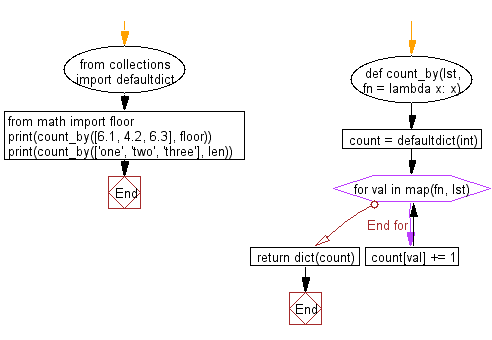﻿ Python: Count of elements in each group from list based groups of elements - w3resource# Python: Count of elements in each group from list based groups of elements

## Python List: Exercise - 216 with Solution

Write a Python program to group the elements of a list based on the given function and returns the count of elements in each group.

• Use collections.defaultdict to initialize a dictionary.
• Use map() to map the values of the given list using the given function./li>
• Iterate over the map and increase the element count each time it occurs.

Sample Solution:

Python Code:

``````from collections import defaultdict
def count_by(lst, fn = lambda x: x):
count = defaultdict(int)
for val in map(fn, lst):
count[val] += 1
return dict(count)
from math import floor
print(count_by([6.1, 4.2, 6.3], floor))
print(count_by(['one', 'two', 'three'], len))
```
```

Sample Output:

```{6: 2, 4: 1}
{3: 2, 5: 1}
```

Flowchart:## Visualize Python code execution:

The following tool visualize what the computer is doing step-by-step as it executes the said program:

Python Code Editor:

Have another way to solve this solution? Contribute your code (and comments) through Disqus.

What is the difficulty level of this exercise?

Test your Python skills with w3resource's quiz

﻿

## Python: Tips of the Day

Floor Division:

When we speak of division we normally mean (/) float division operator, this will give a precise result in float format with decimals.

For a rounded integer result there is (//) floor division operator in Python. Floor division will only give integer results that are round numbers.

```print(1000 // 300)
print(1000 / 300)```

Output:

```3
3.3333333333333335```